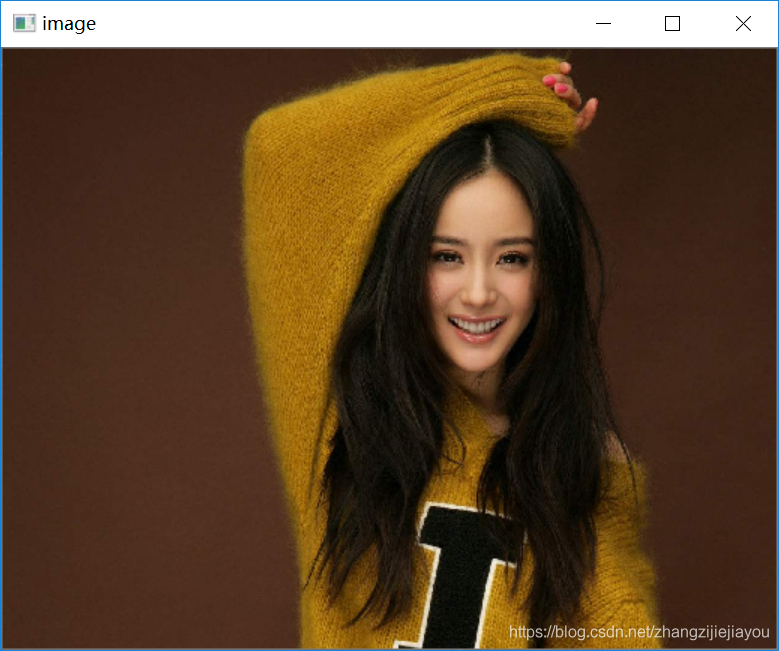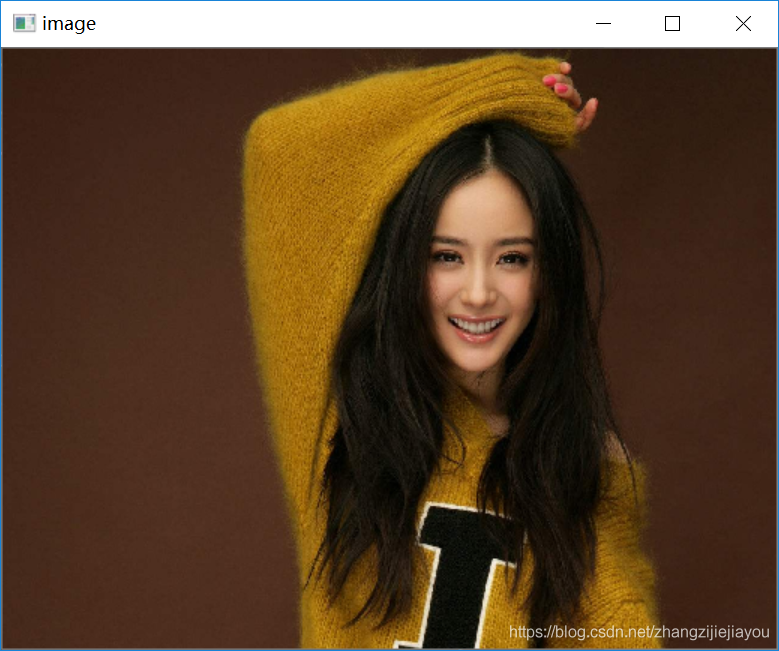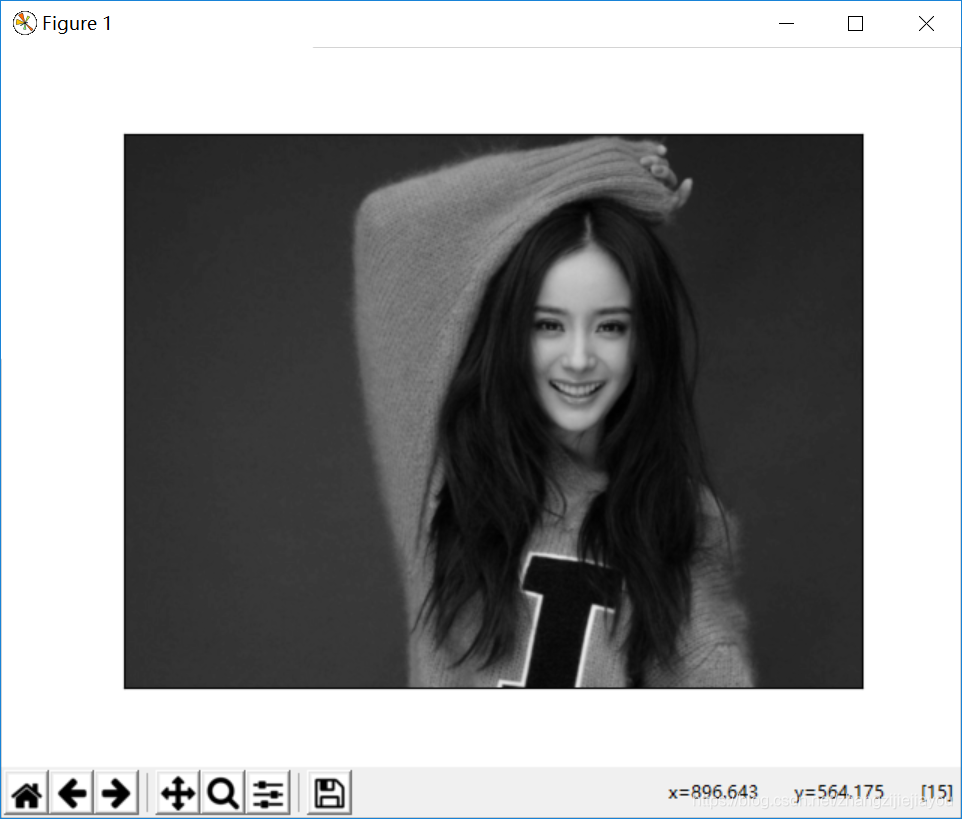Python- 计算机视觉 -OpenCV-ImageAldeo学习目标

import numpy as npimport cv2 # Load an color image in grayscaleimg = cv2.imread('messi5.jpg',0)

Display an image 显示图像

aitkey ()是一个键盘绑定函数。 其参数是以毫秒为单位的时间。 该函数等待任何键盘事件指定的毫秒。 如果您在这段时间内按下任何键，程序将继续运行。 如果 0 被传递，它将无限期地等待一次敲击键。 它也可以设置为检测特定的按键，例如，如果按下键 a 等，我们将在下面讨论。

Destroyallwindows ()只会破坏我们创建的所有窗口。 如果您想要销毁任何特定的窗口，可以使用函数 cv2.destroyWindow () ，在该函数中将确切的窗口名称作为参数传递。Sum it up 总结一下

import numpy as npimport cv2 img = cv2.imread('messi5.jpg',0)cv2.imshow('image',img)k = cv2.waitKey(0)if k == 27:         # wait for ESC key to exit    cv2.destroyAllWindows()elif k == ord('s'): # wait for 's' key to save and exit    cv2.imwrite('messigray.png',img)    cv2.destroyAllWindows()

拓展-使用 Matplotlib

Matplotlib 是 Python 的绘图库，它提供了各种各样的绘图方法。 你会在接下来的文章中看到它们。 在这里，您将学习如何使用 Matplotlib 显示图像。 你可以使用 Matplotlib 缩放图片，保存图片等。

pip install matplotlib

import numpy as npimport cv2from matplotlib import pyplot as plt img = cv2.imread('messi5.jpg',0)plt.imshow(img, cmap = 'gray', interpolation = 'bicubic')plt.xticks([]), plt.yticks([])  # to hide tick values on X and Y axisplt.show()Matplotlib 有很多可供选择的绘图方法。 更多细节请参考 Matplotlib 文档。 一些，我们会在路上看到。

Opencv 加载的彩色图像处于 BGR 模式。 但是 Matplotlib 显示为 RGB 模式。 因此，在 Matplotlib，如果使用 OpenCV 读取图像，彩色图像将无法正确显示。 详情请参阅练习。评论